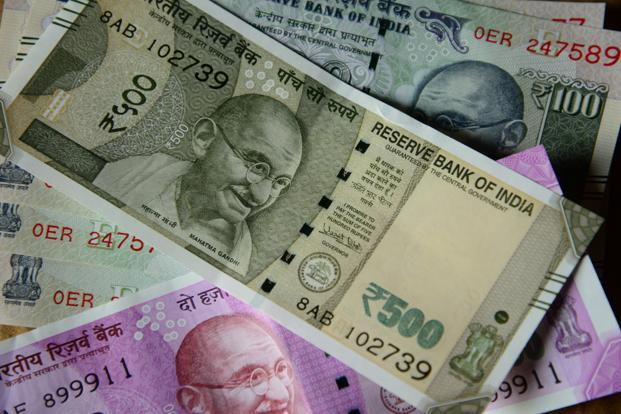# C Program To Count The Total Number Of Notes In A Amount | C Programs

C program to count the total number of notes in a given amount – In this article, we will brief in on the method to count the total number of notes in a given amount.

Suitable examples and sample programs have also been added so that you can understand the whole thing very clearly. The compiler has also been added with which you can execute it yourself.

The method used in this piece is the usual Standard Method.

Cash is considered to be the pinnacle of humanisation and dehumanisation at once.In a particular amount of money, it is natural that there might be a few notes of same or different values.

As far as the Indian currency is concerned, we can see that there are these denominations legally available now to the public. Those are:

• 2000
• 500
• 200
• 100
• 50
• 20
• 10
• 5
• 2
• 1

So, a given amount of money can have either of these denominations depending on the amount in the first place.

Thus, knowing the same in C programming is as follows:

## Using Standard Method

1)The array “n” contains the values of standard notes(i.e 500,100,50,20,10,5,2,1).

2)Read the entered amount and store it in the variable “amount”.

3)for loop iterates with the structure for(i=0;i<8;i++)The amount divided by n[

a)The amount divided by n[i], then the coefficient represents the number of n[i] notes in the given amount. So print the coefficient value.

b)Now amount=remainder of amount/n[i].

Repeat these a,b steps until i<8.

x

## C Program To Print Number Of Days In A Month | Java Tutoring

C program to input the month number and print the number of days in that ...